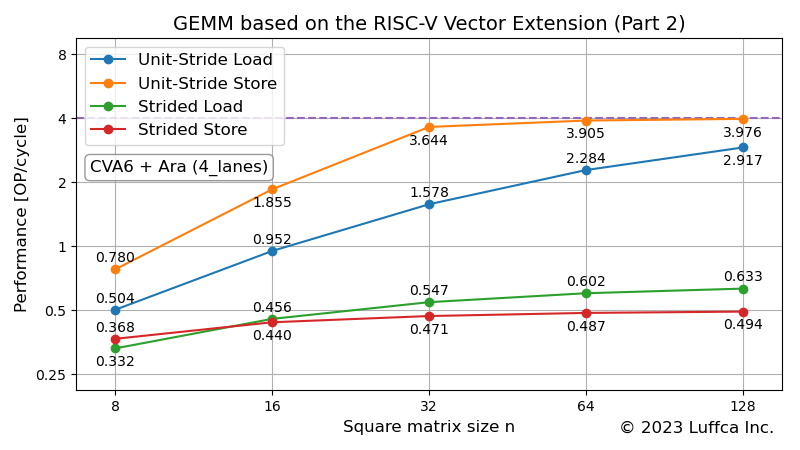# GEMM based on the RISC-V Vector Extension (Part 2)We will describe several times floating-point matrix multiplication kernels based on the RISC-V Vector Extension. In Part 2, we have evaluated vector load/store performance to support transposed matrix using Ara’s RTL simulator.

In the related article, GEMM based on the RISC-V Vector Extension (Part 1), we have evaluated the performance of double-, single- and half-precision floating-point matrix multiplication kernels for arbitrary matrix sizes.

The RISC-V Vector Extension has the following vector load/store instructions for moving values between vector registers and memory.

• Vector Unit-Stride Instructions
• Vector Strided Instructions
• Vector Indexed Instructions

This article describes vector unit-stride instructions for consecutive accesses and vector strided instructions for strided accesses.

### Vector Unit-Stride Instructions

Vector unit-stride instructions move values between consecutive data in memory and vector registers.

```# Vector unit-stride loads and stores

# vd destination, rs1 base address, vm is mask encoding (v0.t or <missing>)
vle8.v    vd, (rs1), vm #  8-bit unit-stride load
vle16.v   vd, (rs1), vm # 16-bit unit-stride load
vle32.v   vd, (rs1), vm # 32-bit unit-stride load
vle64.v   vd, (rs1), vm # 64-bit unit-stride load

# vs store data, rs1 base address, vm is mask encoding (v0.t or <missing>)
vse8.v    vs, (rs1), vm #  8-bit unit-stride store
vse16.v   vs, (rs1), vm # 16-bit unit-stride store
vse32.v   vs, (rs1), vm # 32-bit unit-stride store
vse64.v   vs, (rs1), vm # 64-bit unit-stride store
```

In the example below, vl consecutive 32-bit values from address a are loaded into vector registers v0 and v1.

```float* a;
asm volatile("vsetvli %0, %1, e32, m2, ta, ma" : "=r"(vl) : "r"(n));
asm volatile("vle32.v v0, (%0)" ::"r"(a));
```

### Vector Strided Instructions

Vector strided instructions move values between spaced intervals of data in memory and vector registers.

```# Vector strided loads and stores

# vd destination, rs1 base address, rs2 byte stride
vlse8.v   vd, (rs1), rs2, vm #  8-bit strided load
vlse16.v  vd, (rs1), rs2, vm # 16-bit strided load
vlse32.v  vd, (rs1), rs2, vm # 32-bit strided load
vlse64.v  vd, (rs1), rs2, vm # 64-bit strided load

# vs store data, rs1 base address, rs2 byte stride
vsse8.v   vs, (rs1), rs2, vm #  8-bit strided store
vsse16.v  vs, (rs1), rs2, vm # 16-bit strided store
vsse32.v  vs, (rs1), rs2, vm # 32-bit strided store
vsse64.v  vs, (rs1), rs2, vm # 64-bit strided store
```

In the example below, vl 32-bit values spaced n from address a are loaded into vector registers v0 and v1.

```float* a;
asm volatile("vsetvli %0, %1, e32, m2, ta, ma" : "=r"(vl) : "r"(m));
asm volatile("vlse32.v v0, (%0), %1" ::"r"(a), "r"(n * sizeof(float)));
```

## Vector Load/Store on Ara RTL Simulator

Featured image shows the performance of 32-bit unit-stride load/store (`vle32.v` and `vse32.v`) and strided load/store (`vlse32.v` and `vsse32.v`) in `4_lanes` configuration of Ara’s RTL simulator.

Note that the AXI data width for Ara’s `4_lanes` configuration is 128-bit, so the roofline for 32-bit data is 4.

It can be seen that the unit-stride load/store performance is asymptotic to the roofline, while the strided load/store performance is poor. When the square matrix size is 128, the performance of strided load/store is about 1/4 and 1/8 of that of unit-stride load/store, respectively.

## GEMM transposed matrix support

Here we consider transposed matrix support for GEMM (GEneral Matrix-to-matrix Multiply).

Switching the vector load instruction from unit-stride load to strided load is the easiest way to support transposed matrix in GEMM, but the performance is greatly reduced. The vector load instruction is used in the innermost loop of the GEMM. For this reason, switching to strided load, which has lower performance than unit-stride load, reveals previously hidden memory accesses and affects the number of cycles.

In this case, we chose to create a pre-transposed matrix BT when we need to transpose the matrix B, and then perform unit-stride load from the matrix BT in the innermost loop of the GEMM. This does not degrade the performance of the innermost loop of GEMM, and strided access is only used when creating matrix BT.

There are two possible ways to create the matrix BT using the vector load/store instructions.

• Method 1: Strided store after unit-stride load
• Method 2: Unit-stride store after strided load

Comparing the two methods based on the individual performance shown in the featured image, method 2 has higher performance, and the same results were obtained in actual measurements. When the square matrix size n is 128, the number of cycles to create a single-precision floating-point matrix BT is approximately 30k (≈2n2).

It is also possible to create the matrix BT using the CVA6 processor, but the performance is about 1/4 of method 2.

## Summary

We have evaluated vector load/store performance using Ara’s RTL simulator for transposed matrix support of floating-point matrix multiplication kernels based on the RISC-V Vector Extension.

In Ara, we found that strided access performed poorly compared to sequential access. We chose to create a pre-transposed matrix BT to reduce the number of strided accesses used when we need to transpose the matrix B.

Previous article

Next article